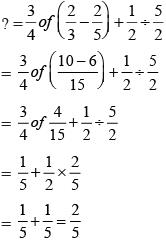# Olympiad Test : Fractions - 2

## 20 Questions MCQ Test Maths Olympiad Class 6 | Olympiad Test : Fractions - 2

Description
This mock test of Olympiad Test : Fractions - 2 for Class 6 helps you for every Class 6 entrance exam. This contains 20 Multiple Choice Questions for Class 6 Olympiad Test : Fractions - 2 (mcq) to study with solutions a complete question bank. The solved questions answers in this Olympiad Test : Fractions - 2 quiz give you a good mix of easy questions and tough questions. Class 6 students definitely take this Olympiad Test : Fractions - 2 exercise for a better result in the exam. You can find other Olympiad Test : Fractions - 2 extra questions, long questions & short questions for Class 6 on EduRev as well by searching above.
QUESTION: 1

### What is the value of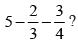Solution:

Required value =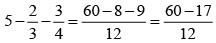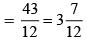QUESTION: 2

### What is the difference of 7/8 and 5/12?

Solution:

Required value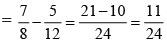QUESTION: 3

### What value is obtained when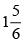is subtracted  from 8?

Solution:

Required value =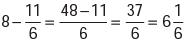QUESTION: 4

What is the equivalent fraction of 3/5 having numerator 21?

Solution: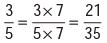QUESTION: 5

What is the equivalent fraction of 5/12 having denominator 84?

Solution: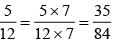QUESTION: 6

What is the equivalent fraction of 56/70 with numerator 4?

Solution: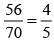QUESTION: 7

What of the following is correct?

Solution: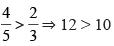QUESTION: 8

What of the following is not correct?

Solution:
QUESTION: 9

A piece of wire is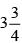m long broke into two pieces. One piece is 5/8 m long, then what is the length of other piece?

Solution:

Required length =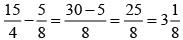QUESTION: 10

What should be added to 29/3 to get 40?

Solution:

Here,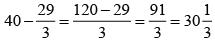QUESTION: 11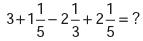Solution: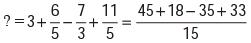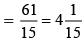QUESTION: 12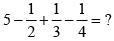Solution: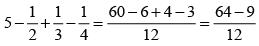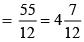QUESTION: 13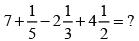Solution: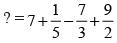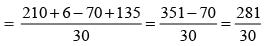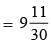QUESTION: 14

What of the following is correct?

Solution: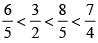QUESTION: 15

What should be added to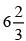to get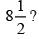Solution: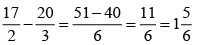QUESTION: 16

What should be subtracted from 5 to get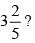Solution: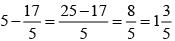QUESTION: 17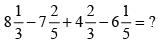Solution: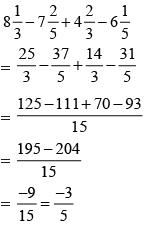QUESTION: 18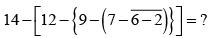Solution:

Required expression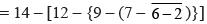= 14 – [12 – {9 – (7 – 4)}]
= 14 – [12 – {9 – 3}]
= 14 – [12 – 6]
= 14 – 6 = 8

QUESTION: 19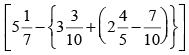Solution:

Given expression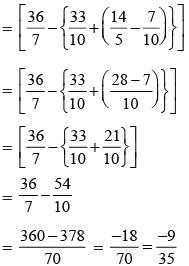QUESTION: 20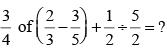Solution: